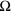# Power and Energy

Next Page: AC, DC and Electrical Signals

Also See: Voltage and Current

## What is power?

Power is the rate of using or supplying energy:

 Power = Energy Time

Power is measured in watts (W)
Energy is measured in joules (J)
Time is measured in seconds (s)

Electronics is mostly concerned with small quantities of power, so the power is often measured in milliwatts (mW), 1mW = 0.001W. For example an LED uses about 40mW and a bleeper uses about 100mW, even a lamp such as a torch bulb only uses about 1W.

The typical power used in mains electrical circuits is much larger, so this power may be measured in kilowatts (kW), 1kW = 1000W. For example a typical mains lamp uses 60W and a kettle uses about 3kW.

## Calculating power using current and voltage

### The equations

 Power = Current × Voltage

There are three ways of writing an equation for power, current and voltage:

 P = I × V
 I = P V
 V = P I

where:

P = power in watts (W)
V = voltage in volts (V)
I  = current in amps (A)

or:

P = power in milliwatts (mW)
V = voltage in volts (V)
I  = current in milliamps (mA)

### The PIV triangle

 P I    V

You can use the PIV triangle to help you remember these three equations. Use it in the same way as the Ohm's Law triangle:

• To calculate power, P: put your finger over P, this leaves I V, so the equation is P = I × V
• To calculate current, I: put your finger over I, this leaves P over V, so the equation is I = P/V
• To calculate voltage, V: put your finger over V, this leaves P over I, so the equation is V = P/I

The amp is quite large for electronics so we often measure current in milliamps (mA) and power in milliwatts (mW).

1mA = 0.001A and 1mW = 0.001W.

## Calculating power using resistance

### The equations

Using Ohm's Law V = I × R

we can convert P = I × V to:

 P = I² × R

and

 P = V² R

where:

P = power in watts (W)
I  = current in amps (A)
R = resistance in ohms ()
V = voltage in volts (V)

### The triangles

You can use triangles to help with these equations too:

 P I²   R

and

 V² P   R

## Wasted power and overheating

Normally electric power is useful, making a lamp light or a motor turn for example. However, electrical energy is converted to heat whenever a current flows through a resistance and this can be a problem if it makes a device or wire overheat. In electronics the effect is usually negligible, but if the resistance is low (a wire or low value resistor for example) the current can be sufficiently large to cause a problem.

You can see from the equation P = I² × R that for a given resistance the power depends on the current squared, so doubling the current will give 4 times the power.

Resistors are rated by the maximum power they can have developed in them without damage, but power ratings are rarely quoted in parts lists because the standard ratings of 0.25W or 0.5W are suitable for most circuits. Further information is available on the resistors page.

Wires and cables are rated by the maximum current they can pass without overheating. They have a very low resistance so the maximum current is relatively large. For further information about current rating please see the cables page.

## Energy

The amount of energy used (or supplied) depends on the power and the time for which it is used:

 Energy = Power × Time

A low power device operating for a long time can use more energy than a high power device operating for a short time.

#### For example:

• A 60W lamp switched on for 8 hours uses 60W × 8 × 3600s = 1728kJ.
• A 3kW kettle switched on for 5 minutes uses 3000W × 5 × 60s = 900kJ.

The standard unit for energy is the joule (J), but 1J is a very small amount of energy for mains electricity so kilojoule (kJ) or megajoule (MJ) are sometimes used in scientific work.

In the home we measure electrical energy in kilowatt-hours (kWh), often just called a 'unit' of electricity when the context is clear. 1kWh is the energy used by a 1kW power appliance when it is switched on for 1 hour:

 1kWh = 1kW × 1 hour

#### For example:

• A 60W lamp switched on for 8 hours uses 0.06kW × 8 = 0.48kWh.
• A 3kW kettle switched on for 5 minutes uses 3kW × 5/60 = 0.25kWh.

You may need to convert the kWh domestic unit to the scientific energy unit, the joule (J):

1kWh = 1kW × 1 hour = 1000W × 3600s = 3.6MJ

Next Page: AC & DC Signals | Study